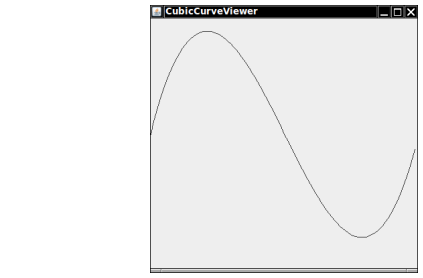It is easy and fun to draw graphs of curves with the Java graphics library. Simply draw 100 line segments joining the points (x, f(x)) and (x + d, f(x + d)), where x ranges from xmin to xmax and d = (xmax − xmin)/100.

Draw the curve f(x) = 0.00005x3 − 0.03x2 + 4x + 200, where x ranges from 0 to 400 in this fashion.

Here is a sample program output:Complete the following file:

### CubicCurveComponent.java

Use the following file:

CubicCurveViewer.java

```import javax.swing.*;

/**
Test driver class.
*/
public class CubicCurveViewer
{
public static void main(String[] args)
{
JFrame frame = new JFrame();

final int FRAME_WIDTH = 400;
final int FRAME_HEIGHT = 400;

frame.setSize(FRAME_WIDTH, FRAME_HEIGHT);
frame.setTitle("CubicCurveViewer");
frame.setDefaultCloseOperation(JFrame.EXIT_ON_CLOSE);

CubicCurveComponent component
= new CubicCurveComponent(0.00005, -0.03, 4, 200);